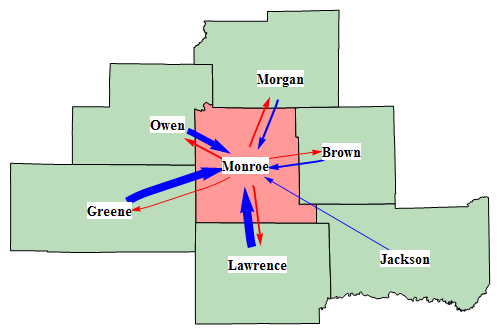## Aug 25, 2009

### Visualize irrational number as random walk

Irrational numbers have decimal expansions that neither terminate nor become periodic. So we can get unlimited “random walk” steps from an irrational numbers.

With the following code, the first 10000 digits of Sqrt is presented as a random walk by converting it in base 4. 0, 1, 2 and 3 digit in base 4 represent 4 directions. Starting point is the green dot, the red one is the ending point.

x = N[Sqrt, 10000];
walk = First@ RealDigits[x, 4];
rn = FoldList[Plus, {0, 0}, {{0, 1}, {1, 0}, {0, -1}, {-1, 0}}[[# + 1]] & /@ walk];
Graphics[{Line[rn], PointSize[Large], Green, Point[First@rn], Red, Point[Last@rn]}]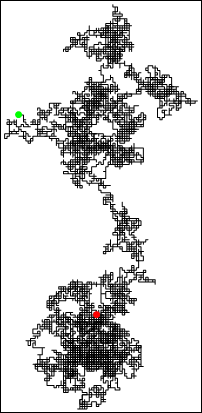We can also display it with ArrayPlot by constructing a sparse array.

(* shift the moves to {1,1} *)

minx = Min[rn[[All, 1]]];
miny = Min[rn[[All, 2]]];
m = # + {-minx + 1, -miny + 1} & /@ rn;

(* sparse array *)

tt = Tally[m];
cd = SparseArray[tt[[All, 1]] -> tt[[All, 2]]];
cd = Transpose[cd];
ArrayPlot[cd, ColorFunction -> "Rainbow", DataReversed -> True, ColorRules -> {0 -> White}]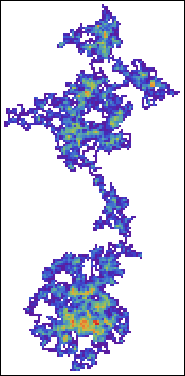Let’s visualize Sqrt, e, Pi in their first 50000 digits. It seems there are some similarities among these images.

Sqrt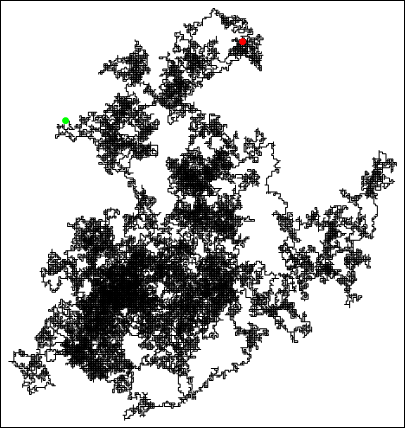Pi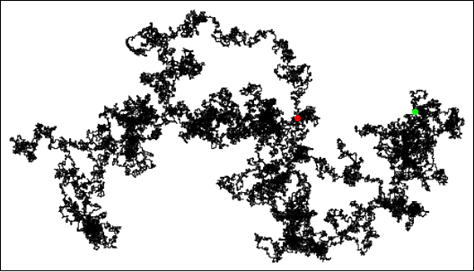e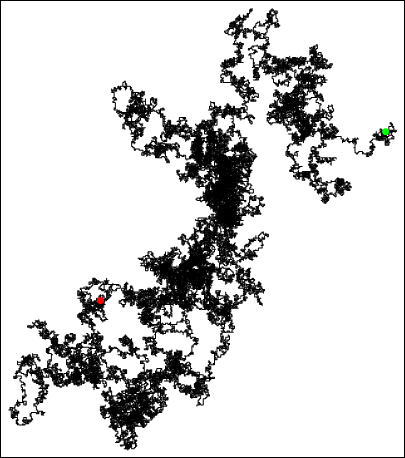Update:

Here's a 3D version:

x = N[Sqrt, 10000];
walk = First@RealDigits[x, 6];
rn = FoldList[ Plus, {0, 0, 0}, {{0, 1, 0}, {1, 0, 0}, {0, -1, 0}, {-1, 0, 0}, {0, 0, 1}, {0, 0, -1}}[[# + 1]] & /@ walk ];
Graphics3D[{Line[rn], PointSize[Large], Green, Point[First@rn], Red, Point[Last@rn]}]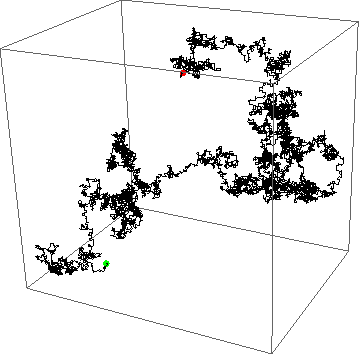You can also try non-irrational number, e.g. 121/5^10

Thanks.

Update 2:

Visualize genome sequence. I know nothing about it, it probably totally meaningless.

GenePlot[g_] := Module[{cs, rn}, walk = Characters[GenomeData [g, "FullSequence"]] /. {"A" -> 1,  "T" -> 2, "G" -> 3, "C" -> 4};
rn = FoldList[Plus, {0, 0}, {{0, 1}, {1, 0}, {0, -1}, {-1, 0}}[[#]] & /@ walk];
Graphics[{Line[rn], PointSize[Large], Green, Point[First@rn], Red, Point[Last@rn]}, Frame -> True, FrameTicks -> None] ]

GenePlot["DDK4"]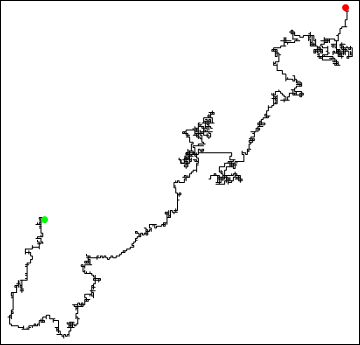GenePlot["DKK3"]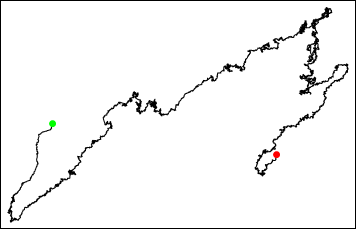## Aug 12, 2009

### Display a plot by clicking a button

Ok, this seems to be very easy in Mathematica.

Button["Show me a plot", Show[Plot[Sin[x], {x, -Pi, Pi}]]]

Then you click the button, nothing happens.

Button["Show me a plot", Print[Plot[Sin[x], {x, -Pi, Pi}]]]

This line will do the job. Or use the following line:

Show[Plot[Sin[x], {x, -Pi, Pi}],
DisplayFunction -> (Button["Show me a plot", Print[#]] &)]

The key here is to use “Print” rather than “Show”. It isn’t clearly explained in the “ref/Button”.

## Aug 7, 2009

### View weighted graph with GraphPlot

Here is a simple example on how to customizing Graphplot. We like to use GraphPlot to visualize the number of people who commute into or out Monroe county from/to its neighbor counties.

g={{"Owen" -> "Monroe", 2813}, {"Greene" -> "Monroe",
3788}, {"Lawrence" -> "Monroe", 4022}, {"Jackson" -> "Monroe",
85}, {"Brown" -> "Monroe", 689}, {"Morgan" -> "Monroe",
821}, {"Monroe" -> "Owen", 676}, {"Monroe" -> "Greene",
207}, {"Monroe" -> "Lawrence", 679}, {"Monroe" -> "Brown",
303}, {"Monroe" -> "Morgan", 617}}

vercoor={"Monroe" -> {-86.529, 39.1621}, "Owen" -> {-86.7642, 39.2868}, "Greene" -> {-86.9403, 39.0246},  "Lawrence" –> {  -86.4923,  38.8627}, "Jackson" -> {-86.0462, 38.8798},  "Brown" -> {-86.2382, 39.203}, "Morgan" -> {-86.4238, 39.4233}}

First try:

GraphPlot[g, VertexLabeling -> True, VertexCoordinateRules -> vercoor]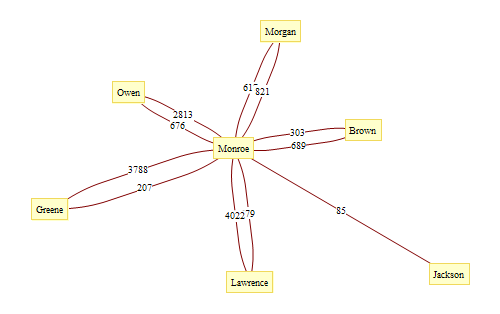Using arrow to indicate in/out seems to be a good idea. We use EdgeRenderingFunction in second try:

GraphPlot[g, VertexLabeling -> True,
EdgeRenderingFunction -> (Arrow[#1, 0.05] &),
VertexCoordinateRules -> vercoor]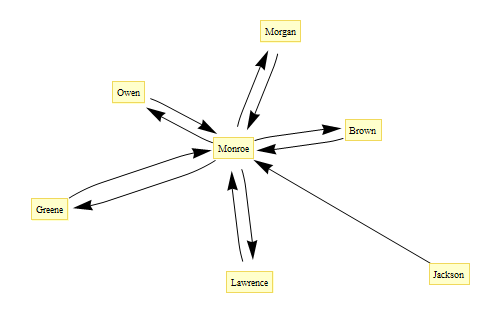However, the labels on the edge is lost. We can handle it in EdgeRenderingFunction.

GraphPlot[g, VertexLabeling -> True,
EdgeRenderingFunction -> ({Text[#3, Mean[#1]], Arrow[#1, 0.05]} &),  VertexCoordinateRules -> vercoor]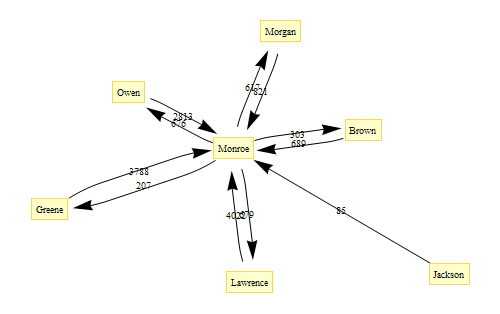The graph is still difficult to read, the commuting pattern isn’t clear at a glance. We further update EdgeRenderingFunction and use the line color and thickness to show the pattern.

GraphPlot[g,
EdgeRenderingFunction -> ({If[#2[] == "Monroe", Red, Blue],
AbsoluteThickness[0.5 + #3/500], Arrowheads[0.02 + #3/120000],  Arrow[#1, 0.05]} &), VertexLabeling -> True,
VertexCoordinateRules -> vercoor]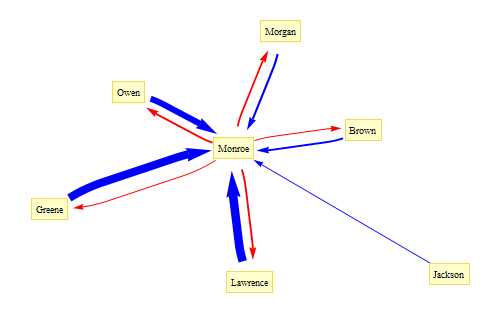In the last try, we use VertexRenderingFunction to make the label more clear.

GraphPlot[g,
EdgeRenderingFunction -> ({If[#2[] == "Monroe", Red, Blue],
AbsoluteThickness[0.5 + #3/500], Arrowheads[0.02 + #3/120000], Arrow[#1, 0.06]} &), VertexLabeling -> True,
VertexCoordinateRules -> vercoor,
VertexRenderingFunction -> ({Text[Style[#2, 14, Bold], #2 /. vercoor, Background -> White]} &)]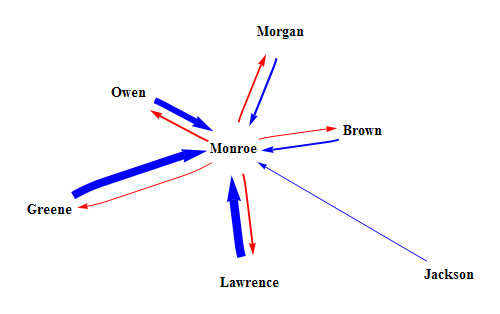Import the shapefile, then you get a map: